# Math in Focus Grade 1 Chapter 9 Practice 2 Answer Key Comparing More Than Two Things

Practice the problems of Math in Focus Grade 1 Workbook Answer Key Chapter 9 Practice 2 Comparing More Than Two Things to score better marks in the exam.

## Math in Focus Grade 1 Chapter 9 Practice 2 Answer Key Comparing More Than Two Things

Look at the picture. Fill in the blanks with the correct names.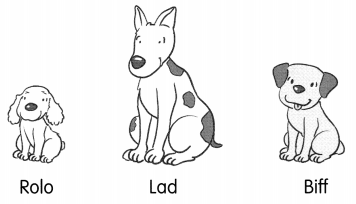Question 1.
____ is taller than Biff.

Question 2.
Biff is taller than ____
Answer: Biff is taller than Rolo

Question 3.
So, Lad is also taller than ____

Then draw the tails on the mouse and dog.

Question 4.
The mouse’s tail is longer than the cat’s tail. The cat’s tail is longer the dog’s tail. So, the mouse’s tail is longer than the dog’s tail.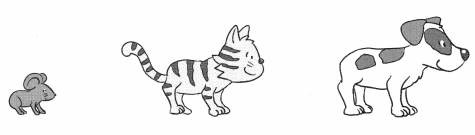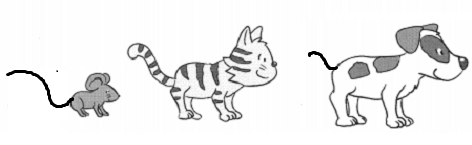Color.
Example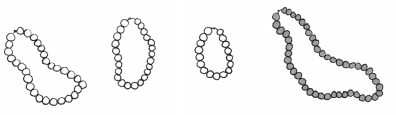Question 5.
the shortest vegetable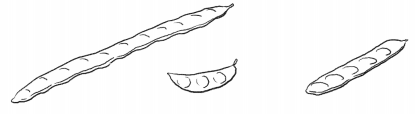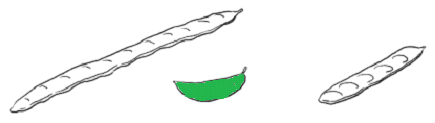Question 6.
the girl with the longest hair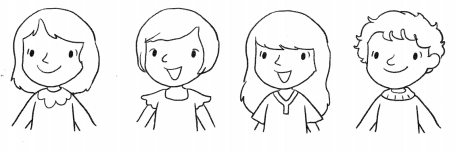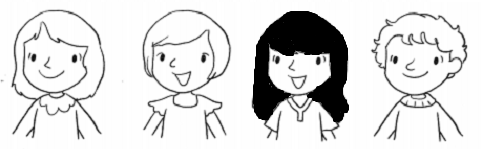Question 7.
the tallest animal.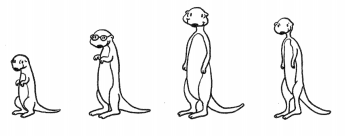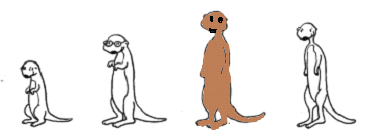Fill in the blanks with taller, tallest, shorter, or shortest.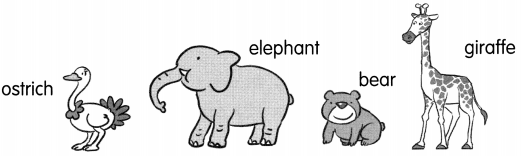Question 8.
The giraffe is the ____ animal.
Answer: The giraffe is the tallest animal.

Question 9.
The ostrich is ___ than the bear.
Answer: The ostrich is taller than the bear.

Question 10.
The ___________ animal is the bear.
Answer: The shortest animal is the bear.

Question 11.
The ostrich is ____ than the elephant.
Answer: The ostrich is shorter than the elephant.

Look at the picture. Fill in the blanks.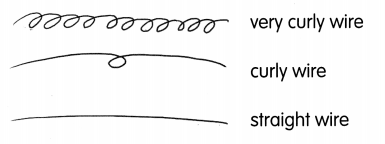Question 12.
The ___________________ is longer than the curly wire.
Answer: The very curly hair is longer than the curly wire.

Question 13.
The curly wire ¡s longer than the _______.
Answer: The curly wire ¡s longer than the straight hair.

Question 14.
The ___________________ is the longest wire.
Answer: The very curly hair is the longest wire.